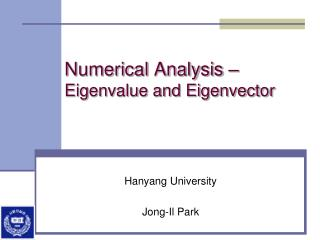DownloadDownload PresentationNumerical Analysis – Eigenvalue and Eigenvector

# Numerical Analysis – Eigenvalue and Eigenvector

Télécharger la présentation## Numerical Analysis – Eigenvalue and Eigenvector

- - - - - - - - - - - - - - - - - - - - - - - - - - - E N D - - - - - - - - - - - - - - - - - - - - - - - - - - -
##### Presentation Transcript

1. Numerical Analysis – Eigenvalue and Eigenvector Hanyang University Jong-Il Park

2. Eigenvalue problem : eigenvalue x : eigenvector ※ spectrum: a set of all eigenvalue

3. Eigenvalue • Eigenvalue if det  x=0(trivial solution) To obtain a non-trivial solution, det  ;Characteristic equation

4. Properties of Eigenvalue 1) Trace 2) det 3) If A is symmetric, then the eigenvectors are orthogonal: 4) Let the eigenvalues of then, the eigenvalues of (A - aI)

5. Geometrical Interpretation of Eigenvectors • Transformation : The transformation of an eigenvector is mapped onto the same line of. • Symmetric matrix  orthogonal eigenvectors • Relation to Singular Value if A is singular  0 {eigenvalues}

6. Eg. Calculating Eigenvectors(I) • Exercise 1) ; symmetric, non-singular matrix ( = -1, -6) 2) ; non-symmetric, non-singular matrix ( = -3, -4)

7. Eg. Calculating Eigenvectors(II) 3) ; symmetric, singular matrix ( = 5, 0) 4) ; non-symmetric, singular matrix ( = 7, 0)

8. Discussion • symmetric matrix => orthogonal eigenvectors • singular matrix => 0 {eigenvalue} • Investigation into SVD

9. Similar Matrices • Eigenvalues and eigenvectors of similar matrices Eg. Rotation matrix

10. Similarity Transformation • Coordinate transformation • x’=Rx, y’=Ry • Similarity transformation • y=Ax • y’=Ry=RAx=RA(R-1 x’)= RAR-1 x’=Bx’ B = RAR-1

11. Numerical Methods(I) • Power method • Iteration formula • for obtaining large

12. Eg. Power method

13. Numerical Methods (II) • Inverse power method • Iteration formula • for obtaining small

14. Exploiting shifting property Let the eigenvalues of then, the eigenvalues of (A - aI) • Finding the maximum eigenvalue with opposite sign after obtaining • Accelerating the convergence when an approximate eigenvalue is available

15. Deflated matrices • It is possible to obtain eigenvectors one after another • Properly assigning the vector x is important • Eg. Wielandt’s deflation

16. Eg. Using Deflation(I)

17. Eg. Using Deflation(II)

18. Numerical Methods (III) • Hotelling's deflation method • Iteration formula: given • for symmetric matrices • deflation from large to small

19. Numerical Methods (IV) • Jacobi transformation • Successive diagonalization without changing . • for symmetric matrices

20. Homework #7 • Generate a 9x9 symmetric matrix A by using random number generator(Gaussian distribution with mean=0 and standard deviation=1.1]). Then, compute all eigenvalues and eigenvectors of A using the routines in the book, NR in C. Print the eigenvalues and their corresponding eigenvectors in the descending order. • You may use • jacobi(): Obtaining eigenvalues using the Jacobi transformation • eigsrt(): Sorting the results of jacobi() [Due: Nov. 19]